# Cruise control

Jump to: navigation, search

This page documents the cruise control system that is used as a running example throughout the text. A detailed description of the dynamics of this system are presented in Chapter 3 - Examples. This page contains a description of the system, including the models and commands used to generate some of the plots in the text.

## Introduction

Cruise control is the term used to describe a control system that regulates the speed of an automobile. Cruise control was commercially introduced in 1958 as an option on the Chrysler Imperial. The basic operation of a cruise controller is to sense the speed of the vehicle, compare this speed to a desired reference, and then accelerate or decelerate the car as required. The figure to the right shows a block diagram of this feedback system.

A simple control algorithm for controlling the speed is to use a "proportional plus integral" feedback. In this algorithm, we choose the amount of gas flowing to the engine based on both the error between the current and desired speed, and the integral of that error. The plot on the right shows the results of this feedback for a step change in the desired speed and a variety of different masses for the car (which might result from having a different number of passengers or towing a trailer). Notice that independent of the mass (which varies by a factor of 3), the steady state speed of the vehicle always approaches the desired speed and achieves that speed within approximately 5 seconds. Thus the performance of the system is robust with respect to this uncertainty.

## Dynamic model

To develop a mathematical model we start with a force balance for the car body. Letbe the speed of the car,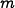the total mass (including passengers),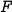the force generated by the contact of the wheels with the road, andthe disturbance force due to gravity, friction and aerodynamic drag. The equation of motion of the car is simply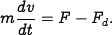The forceis generated by the engine, whose torque is proportional to the rate of fuel injection, which is itself proportional to a control signal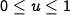that controls the throttle position. The torque also depends on engine speed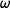. A simple representation of the torque at full throttle is given by the torque curve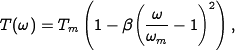Let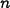be the gear ratio andthe wheel radius. The engine speed is related to the velocity through the expression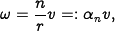and the driving force can be written asTypical values offor gears 1 through 5 are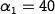,,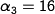,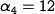and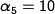. The inverse ofhas a physical interpretation as the effective wheel radius. The figure to the right shows the torque as a function of vehicle speed. The figure shows that the effect of the gear is to "flatten" the torque curve so that an almost full torque can be obtained almost over the whole speed range.

The disturbance forcehas three major components:, the forces due to gravity;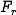, the forces due to rolling friction; and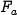, the aerodynamic drag. Letting the slope of the road be, gravity gives the force, whereis the gravitational constant. A simple model of rolling friction is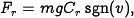whereis the coefficient of rolling friction and sgn() is the sign ofor zero if. A typical value for the coefficient of rolling friction is. Finally, the aerodynamic drag is proportional to the square of the speed:### SIMULINK model

The file cruise_ctrl.mdl contains a nonlinear model of a cruise controller for a car, depicted below.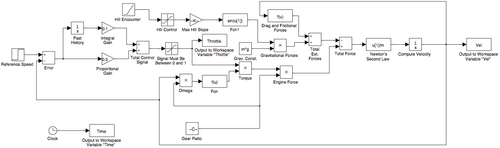The model contains all of the dynamics described in Section 3.1.

### MATLAB model

An alternative model for the system is a MATLAB model suitable for use with the 'ode45' function. The file {{{2}}} contains a nonlinear model of the car dynamics consistent with the description given in Section 3.1.

## MATLAB files

A number of MATLAB files are available that correspond to examples in the printed text. This section contains links to the various files that are available. Note that you will also need some of the MATLAB tools.

Standalone versions of the model:

• cruise_ctrl.mdl - SIMULINK model for cruise controller in Section 3.1
• cruisedyn.m - MATLAB function to compute the acceleration given the vehicle state, inputs and parameters

Linearized models (all files required):

Controller designs (require linearized models):

## Exercises

The following exercises make use of the cruise control model described here: